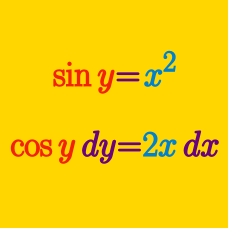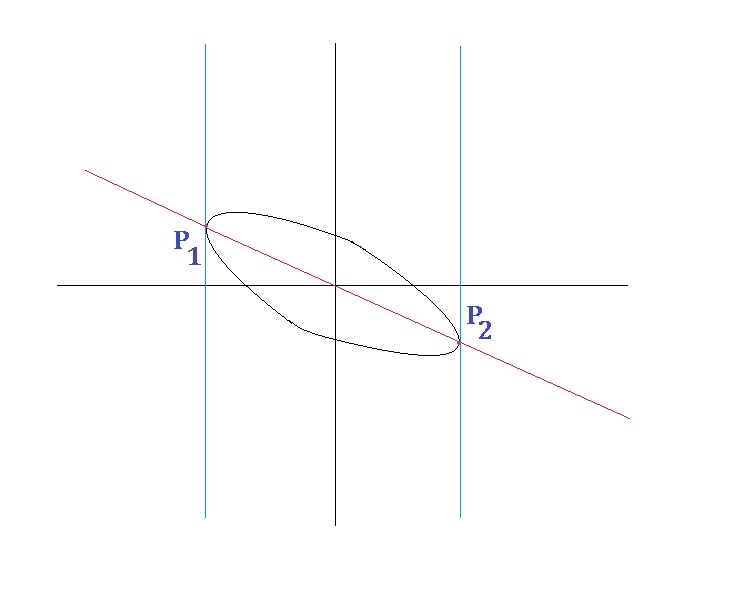Calculus

Implicit Differentiation: Level 3 Challenges

$y=\sqrt { x+\sqrt { x+\sqrt { x+\sqrt { x+\cdots }}}}$

For $x>0$ , define $y$ as above. What is $\dfrac{dy}{dx}$?

• $P: \dfrac{1}{2y-1}$
• $Q: \dfrac{x}{x+2y}$
• $R: \dfrac { 1 }{ \sqrt { 1+4x } }$
• $S: \dfrac{y}{2x + y}$

$\large x^{p}y^{q}=(x+y)^{p+q}$

If the above equation holds true for some reals $x$, $y$, $p$ and $q$, then what is true about $\dfrac {dy}{dx}$?

Find the angle (in degrees) of intersection of the curves

\begin{cases} \begin{aligned} x^{3}-3xy^{2}&=a \\ 3yx^{2}-y^{3}&=b. \end{aligned} \end{cases}

Details and Assumptions:

• $a$ and $b$ are real numbers.
• The angle of intersection of curves is the angle between the tangents to the curves at the point of intersection.

Let $y = \sqrt{x+\sqrt{12x-36}}.$

The value of $\frac{dy}{dx}$ at $x = 4$ can be expressed as $\frac{m}{n},$ where $m$ and $n$ are coprime positive integers.

Find the value of $m+n.$As shown above is the elliptical graph of $x^2 + xy + y^2 = 7$, where $P_{1}$ and $P_{2}$ are the points on the graph with minimum and maximum $x$ coordinates respectively, and the red line $l$ is the linear graph passing through $P_{1}$ and $P_{2}$.

If the tangent parallel to line $l$ touches the graph at a point $P_{3} (a,b)$ for some real numbers $a,b$, compute $a^2 + b^2$.

×